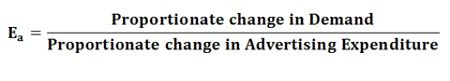# Types of Elasticity of Demand

Definition:
The Elasticity of Demand measures the percentage change in quantity demanded for a percentage change in the price. Simply, the relative change in demand for a commodity as a result of a relative change in its price is called as the elasticity of demand.

## Types of Elasticity of Demand1. Price Elasticity of Demand: The price elasticity of demand, commonly known as the elasticity of demand refers to the responsiveness and sensitiveness of demand for a product to the changes in its price. In other words, the price elasticity of demand is equal toNumerically,Where,
ΔQ = Q1 –Q0, ΔP = P1 – P0, Q1= New quantity, Q2= Original quantity, P1 = New price, P0 = Original priceThe following are the main Types of Price Elasticity of Demand:

• Perfectly Elastic Demand
• Perfectly Inelastic Demand
• Relatively Elastic Demand
• Relatively Inelastic Demand
• Unitary Elastic Demand
2. Income Elasticity of Demand: The income is the other factor that influences the demand for a product. Hence, the degree of responsiveness of a change in demand for a product due to the change in the income is known as income elasticity of demand. The formula to compute the income elasticity of demand is:For most of the goods, the income elasticity of demand is greater than one indicating that with the change in income the demand will also change and that too in the same direction, i.e. more income means more demand and vice-versa.
3. Cross Elasticity of Demand: The cross elasticity of demand refers to the change in quantity demanded for one commodity as a result of the change in the price of another commodity. This type of elasticity usually arises in the case of the interrelated goods such as substitutes and complementary goods. The cross elasticity of demand for goods X and Y can be expressed as:The two commodities are said to be complementary, if the price of one commodity falls, then the demand for other increases, on the contrary, if the price of one commodity rises the demand for another commodity decreases. For example, petrol and car are complementary goods.

While the two commodities are said to be substitutes for each other if the price of one commodity falls, the demand for another commodity also decreases, on the other hand, if the price of one commodity rises the demand for the other commodity also increases. For example, tea and coffee are substitute goods.

4. Advertising Elasticity of Demand:  The responsiveness of the change in demand to the change in advertising or rather promotional expenses, is known as advertising elasticity of demand. In other words, the change in the demand as a result of the change in advertisement and other promotional expenses is called as the advertising elasticity of demand. It can be expressed as:Numerically,Where,
Q1 = Original Demand
Q2= New Demand